# NCERT Solutions for Class 7 Maths Chapter 10 Practical Geometry

NCERT Solutions for Class 7 Maths Chapter 10 Practical Geometry

### NCERT Solutions for Class 7 Maths Chapter 10 Practical Geometry Ex 10.1

NCERT Solutions for Class 7 Maths Chapter 10 Practical Geometry Exercise 10.1
Ex 10.1 Class 7 Maths Question 1.
Draw a line, say AB, take a point C outside it. Through C draw a line parallel to AB using ruler and compasses only.
Solution:
Steps of construction:
(i) Draw a line AB.
(ii) Take any point D on it.
(iii) Join the given point C to D and mark ∠1 to ∠CDB.
(iv) Mark ∠1 = ∠2 at C and produce to both side.
(v) MN is the required line.Using the Property of Alternate Angles

Ex 10.1 Class 7 Maths Question 2.
Draw a line Z. Draw a perpendicular to l at any point on l. On this perpendicular choose a point X, 4 cm away from l. Through X, draw a line m parallel to l.
Solution: ‘Steps of construction:
(i) Draw a given line Z and take any point P on it.
(ii) Draw a perpendicular line at P to the line Z such that PX = 4 cm.(iii) Draw ∠2 = ∠1 i.e. 90° at PX and produce the line both sides.
(iv) m is the required line parallel to Z through X.
Using Properties of Alternate Angles

Ex 10.1 Class 7 Maths Question 3.
Let l be a line and P be a point not on l. Through P, draw a line m parallel to P. Now join P to any point Q on l. Choose any other point R on m. Through R, draw a line parallel to PQ. Let this meet l at S. What shape do the two sets of parallel lines enclose?
Solution:
Steps of Construction:
(i) Draw a line l and take any point P not on l.
(ii) Draw a line m parallel to l through P.
(iii) Join P and Q.
(iv) PQ makes ∠1 with l and ∠2 with m which are equal angles.
(v) Take any point R on m and draw ∠3 equal to ∠ 2 to meet l at S such that PQ || RS.

(vi) Since l || m and PQ || RS. Therefore, PQSR is a parallelogram.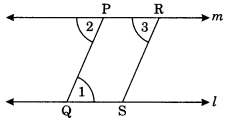Using the properties of parallel lines and transversal line and alternate angles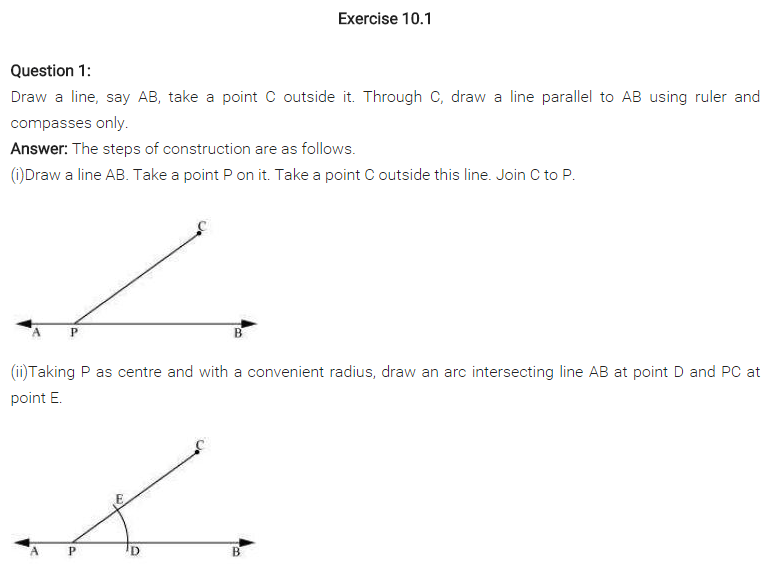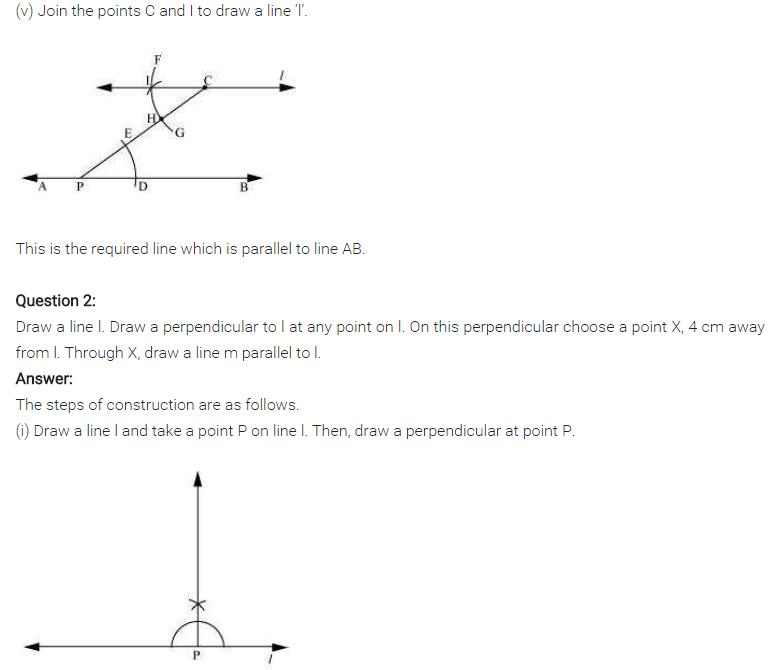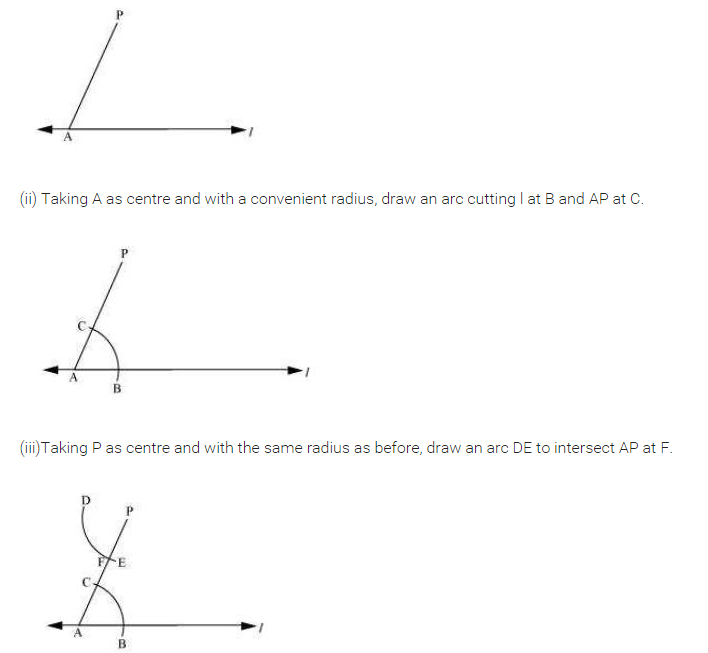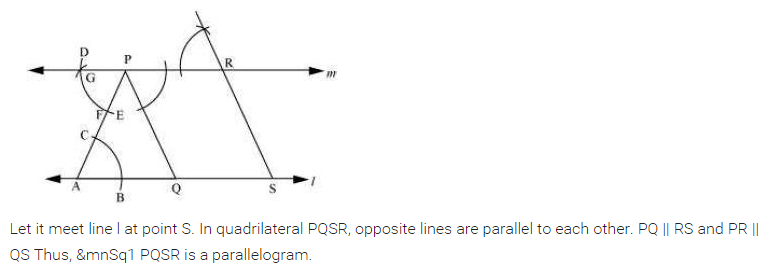## SabDekho

The Complete Educational Website